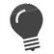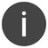# DECR

Decrement a numeric variable

 WTSupported in traditional Synergy on Windows WNSupported in Synergy .NET on Windows USupported on UNIX VSupported on OpenVMS
```DECR variable
```

Arguments

variable

The variable to be decremented. (n)

Discussion

The DECR statement decreases the value of a specified numeric value by 1.

The value of variable can be positive or negative. If the decremented value of variable overflows its size, variable is set to its zero representation. For example, the following code fragment decrements dvar from -9 to 0, because dvar is a d1 field instead of a d2:

```record
dvar        ,d1,    -9
proc
decr dvar
```Using the DECR statement is more efficient than subtracting 1 from a variable.With integer variables, if the variable has the maximum negative value for its size, the following statements have different results:```decr ivar ivar = ivar - 1 ``` In the example below, i1 = i1 – 1 is equivalent to i1 = -129, which results in 127. See Moving integer data to an integer destination for more information. This behavior is consistent with the behavior of decimal variables, which may be used as counters.```record i1 ,i1, -128 ;Max negative value for an i1 field proc decr i1 ;Result is 0 i1 = -128 i1 = i1 - 1 ;Result is 127 ```

INCR statement

Examples

The following example:

```decr dvar(2,5)
```

is functionally equivalent to

`dvar(2,5) = dvar(2,5) - 1`